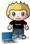# Getting a bounding box from your SQL Geography

posted in: Uncategorized | 0I saw a question posed on twitter last week asking how to pass a SQL 2008 Geography as the bounding box to the many search engines on the web that now allow a spatial query. Flickr would be an example of this, the search api takes a bbox parameter described as:

`bbox` (Optional)

A comma-delimited list of 4 values defining the Bounding Box of the area that will be searched.
The 4 values represent the bottom-left corner of the box and the top-right corner, minimum_longitude, minimum_latitude, maximum_longitude, maximum_latitude.

We had to do this for some SSRS maps we made and thanks to Rob Farley from LobsterPot we created this helper in SQL:

CREATE function [dbo].[GetLatLongBounds](@geo geography) returns table as
return (
select
MIN(@geo.STPointN(n.num).Lat) as MinLat,
MAX(@geo.STPointN(n.num).Lat) as MaxLat,
MIN(@geo.STPointN(n.num).Long) as MinLong,
MAX(@geo.STPointN(n.num).Long) as MaxLong
from dbo.nums n
where n.num <= @geo.STNumPoints()
)
;
GO

This makes us of a table of numbers calls dbo.nums, a trick used widely when you need a list of all indexes:

CREATE TABLE [dbo].[nums](
[num] [int] NOT NULL,
PRIMARY KEY CLUSTERED
(
[num] ASC
)WITH (PAD_INDEX  = OFF, STATISTICS_NORECOMPUTE  = OFF, IGNORE_DUP_KEY = OFF, ALLOW_ROW_LOCKS  = ON, ALLOW_PAGE_LOCKS  = ON) ON [PRIMARY]
) ON [PRIMARY]

GO

Populate the table of numbers with the largest number you will need, we put a million numbers in there.

Use the bounds function like this, where you want the bounds returned for a particular GEO in MYTABLE with MYID of 42:

SELECT b.*

FROM [dbo].[MYTABLE]

CROSS APPLY (SELECT * FROM [dbo].[GetLatLongBounds](g.GEO)) b

WHERE MYID = 42

The output is perfect for your next bounding box search. If you really want to impress, when the results come back why not test their STIntersects() with the Geography and filter out those false positives from the bounding box?

You can reach me on http://twitter.com/soulsolutions